Home > A2C > Chapter 7 > Lesson 7.1.1 > Problem7-11

7-11.
1. Solve each exponential equation for x. Homework Help ✎

1. 10x = 16

2. 10x = 41

3. 3x = 729

4. 10x = 101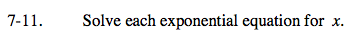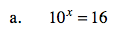Rewrite it in k = logbN format.

Use your calculator for log1016.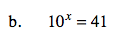See part (a).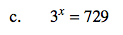What are some powers of 3? 34, 35,...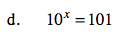See part (a).